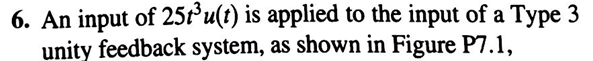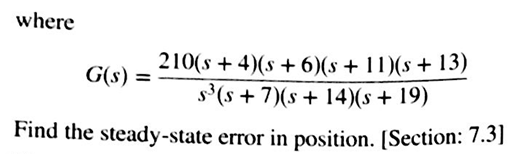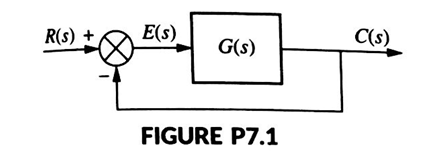# Question 6. An input of 25t’u(t) is applied to the input of a Type 3 unity feedback system, as shown in Figure P7.1, where G(s) = ? G _210(s + 4)(s + 6)(s + 11)(s + 13) S'(s + 7)(s + 14)(s + 19) Find the steady-state error in position. (Section: 7.3 R(S) + G(s) FIGURE P7.1QCHURO The Asker · Electrical EngineeringTranscribed Image Text: 6. An input of 25t’u(t) is applied to the input of a Type 3 unity feedback system, as shown in Figure P7.1, where G(s) = ? G _210(s + 4)(s + 6)(s + 11)(s + 13) S'(s + 7)(s + 14)(s + 19) Find the steady-state error in position. (Section: 7.3 R(S) + G(s) FIGURE P7.1
More
Transcribed Image Text: 6. An input of 25t’u(t) is applied to the input of a Type 3 unity feedback system, as shown in Figure P7.1, where G(s) = ? G _210(s + 4)(s + 6)(s + 11)(s + 13) S'(s + 7)(s + 14)(s + 19) Find the steady-state error in position. (Section: 7.3 R(S) + G(s) FIGURE P7.1